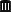## 0.0024 > 0 ■ [RSABE / ABEL]

Hi Sereng,

» […] For two of the studies, we met the bioequivalence criteria for AUC and Cmax. For the third study, we met the bioequivalence criteria for AUC. However, for Cmax, the scaled ABE for was LCmax 95% Upper bound 0.0024.

Bad luck. If studies are powered to 80% and all assumptions are exactly realized, one out of five is expected to fail by pure chance (i.e., the type II error hits). Business as usual.

» There do not appear to be any specific FDA guidance expressly stating the number of significant figures required in the non-zero calculations for RSABE.

Correct.

» In the FDA Draft Guidance, Bioavailability and Bioequivalence Studies for Orally Administered Drug Products – General Considerations (July 2002),…

Where did you find this goody? Even Revision 1 of March 2003 was removed from the FDA’s site and can be found only in theInternet Archive. Both Population and Individual BE were removed in Rev. 1 and only ABE kept.

» … I did find the following language. "If the study estimate of standard deviation is ≤σT0, the constant-scaled criterion and its associated confidence interval should be computed. Otherwise, the reference-scaled criterion and its confidence interval should be computed. If the upper confidence bound for the appropriate criterion is negative or zero, conclude population BE. If the upper bound is positive, do not conclude population BE."

OK, the same text is given in the “Statistical Approaches Establishing BE” of Jan 2001, which seemingly still is in force. However, according to what you wrote above, you are dealing with RSABE, not PBE/IBE.
• The hypotheses in RSABE are $$\small{H_0:(\mu_\textrm{T}/\mu_\textrm{R})^2-\theta_\textrm{s}\cdot s_\textrm{wR}^{2}{\color{Red}{\: >0}}\:vs\:H_1:(\mu_\textrm{T}/\mu_\textrm{R})^2-\theta_\textrm{s}\cdot s_\textrm{wR}^{2}\color{Red}{\: \leq 0}}\tag{1}$$ and $$\small{H_0:100\cdot \mu_\textrm{T}/\mu_\textrm{R}{\color{Red}{\, \notin}}\, \{80.00,125.00\} \:vs\:H_1:100\cdot \mu_\textrm{T}/\mu_\textrm{R}{\color{Red}{\, \subseteq}}\, \{80.00,125.00\}}\tag{2}$$ leaving no room for interpretation. According to the guidances rounding to the second decimal place should be performed only for the second set of hypotheses.
• Only RSABE appears in the ANDA draft guidance of December 2013 (footnote 10 on page 4). The pro­ge­sterone guidance (outlining the details of RSABE) states:
the 95% upper confidence bound for $$\small{\left(\overline{Y_\textrm{T}}-\overline{Y_\textrm{R}}\right)^2-\theta\: \sigma_{\textrm{wR}}^2}$$ must be ≤0

» This is uncharted territory for us. is there any precedence for this?

Duno.

» Should we truncate our results to 0.00?

Because you don’t like it? 0.0024 is positive. Hence, $$\small{H_0}$$ of inequivalence in $$\small{(1)}$$ is not rejected. There is not a single word about rounding of the upper bound in any guidance.
Where would you draw the line? Would you round 0.4999 (commercial) or 0.5 (ISO/IEC/IEEE 60559:2011) down to zero as well?

» Can we conclude BE in our study report?

You can try anything. Technically and acc. to the guidance you failed to demonstrate RSABE.

» If we can conclude BE, should we truncate the Cmax value to 0.00?

What do you mean by that?

Dif-tor heh smusma 🖖 Довге життя Україна!Helmut SchützThe quality of responses received is directly proportional to the quality of the question asked. 🚮
Science QuotesIng. Helmut Schütz